Wednesday 21st October 2020CBSE Guess > Papers > Important Questions > Class XII > 2009 > Maths > Mathematics By Anil Kumar Tondak

CBSE CLASS XII

Three Dimensional Geometry

Q. 1. Find the direction cosines of X, Y and Z-axis.

Q. 2. Find the equation of the line which passes through the point (1, 2, 3) and is parallel to the vector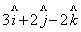.

Q. 3. Find the value of p so that the lines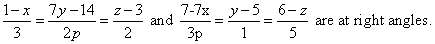Q. 4. Find the shortest distance between the lines whose vector equations are:-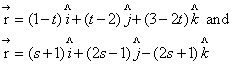Q. 5. Find the coordinates of the foot of the perpendicular drawn from the origin to the plane 2x – 3y + 4z -6 = 0.

Q. 6. Find the vector equation of the plane passing through the intersection of planes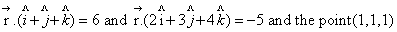Q. 7. Find the angle between the line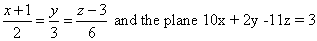Q. 8. Prove that if a plane has the intercepts a, b, c and is at a distance of p units from the origin, then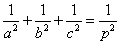Q. 9. Show that the angles between the diagonals of a cube is cos-1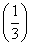.

Q. 10. Find the equation of the line passing through the point (-1, 3, -2) and perpendicular to the lines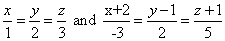Q. 11. Find the foot of the perpendicular drawn from the point (0, 2, 3) on the line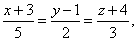Also, find the length of the perpendicular.

Q. 12. Find the shortest distance between the following pairs of lines whose cartesian equations are :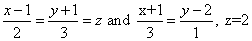Q. 13. A plane meets the coordinate axis in A, B, C such that the centroid of triangle ABC is the point (p,q,r). Show that the equation of the plane is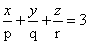.

Q. 14. Find the equation of the plane passing through the point (1, 1, -1) and perpendicular to the planes x + 2y + 3z – 7=0 and 2x – 3y + 4z = 0.

Q. 15. Find the distance between parallel planes,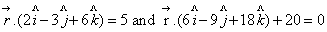Q. 16. Show that the lines :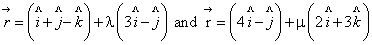are coplanar.
Also, find the plane containing these two lines.

Prepared By Anil Kumar Tondak
M.Sc.(Maths), M.Ed., M.A.(Eco),M.B.A.(Mkt)
Mob No. 9811363962

 Maths